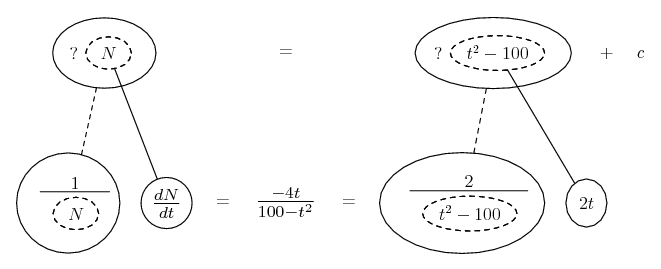# Thread: First order ODE

1. ## First order ODE

Hey,

I'm struggling to solve this first order ODE;

(100-t^2)dN/dt + 4tN = 0, with the initial condition N(0)=10

I got as far as dN/dt = -4tN/(100-t^2), but im not sure where to go from here, or if that is even the right thing to do.

Any suggestions?

Thanks,
Function

2. Good - multiply each side by 1/N. Now you can integrate both sides with respect to t.

Edit:

Just in case a picture helps...... where... is the chain rule. Straight continuous lines differentiate downwards (integrate up) with respect to x, and the straight dashed line similarly but with respect to the dashed balloon expression (which is the inner function of the composite and hence subject to the chain rule).

__________________________________________

Don't integrate - balloontegrate!

http://www.ballooncalculus.org/forum/top.php

Draw balloons with LaTeX: http://www.ballooncalculus.org/asy/doc.html

3. Thanks, by doing that I got an answer of;
N=2log((t^2)-100)+C but i'm not sure if i've done it correctly.
Any thoughts??

Thanks,
Function

4. Log on the left, too - see pic. Then bring the 2 into the log... 2 ln|x| = ln|x^2|... and call C "ln|A|"... and take the exp of both sides

#### Search Tags

ode, order